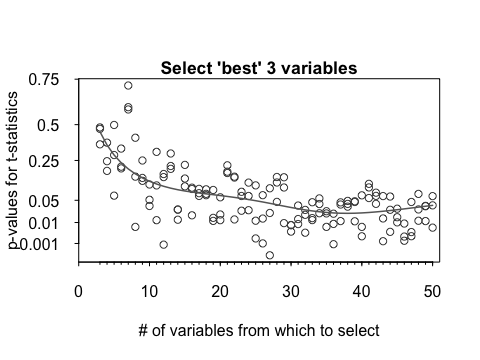# Sampling Properties of Variable Selection

knitr::opts_chunk$set(echo=FALSE) The function varselect() in the leaps package can be used for variable selection. Available approaches are forward, backward, and exhaustive selection. The DAAG package has the functions bestsetNoise() and bsnVaryNvar() that are designed to give insight on the sampling properties of output from the function lm(), when one of these variable selection approaches has been used to choose the explanatory variables that appear in the model. ## $$p$$-value based variable selection, with data that are pure noise The function bestsetNoise() (DAAG) can be used to experiment with the behaviour of various variable selection techniques with data that is purely noise. , Section 6.5, pp.~197-198, gives examples of the use of this function. For example, try: bestsetNoise(m=100, n=40, nvmax=3) bestsetNoise(m=100, n=40, method="backward", nvmax=3) The analyses will typically yield a model that, if assessed using output from the R function lm(), appears to have highly (but spuriously) statistically significant explanatory power, with one or more coefficients that appear (again spuriously) significant at a level of around $$p$$=0.01 or less. The function bestsetNoise() has provision to specify the model matrix. Model matrices with uncorrelated columns of independent Normal data, which is the default, are not a good match to most practical situations. ## $$p$$-values, vs number of variables available for selection As above, datasets of random normal data were created, always with 100 observations and with the number of variables varying between 3 and 50. For three variables, there was no selection, while in other cases the best'' three variables were selected, by exhaustive search. Figure \@ref(fig:exhaust) plots the p-values for the 3 variables that were selected against the total number of variables. The fitted line estimates the median$p\$-value, as a function ofnvar. The functionbsnVaryNvar()that is used for the calculations makes repeated calls tobestsetNoise(). Similar results will be obtained from use of forward or backward selection.Figure 1: $$p$$-values from the R function lm(), versus number of variables available for selection.

Code is:

## Code
suppressMessages(library(quantreg, quietly=TRUE))
library(splines, quietly=TRUE)
set.seed(37)   # Use to reproduce graph that is shown
bsnVaryNvar(m=100, nvar=3:50, nvmax=3)

When all 3 variables are taken, the $$p$$-values are expected to average 0.5. Notice that, for selection of the best 3 variables out of 10, the median $$p$$-value has reduced to about 0.1.

## Reference

Maindonald, J H, and W J Braun. 2011. Data Analysis and Graphics Using r. An Example-Based Approach. 3rd ed. Cambridge University Press.